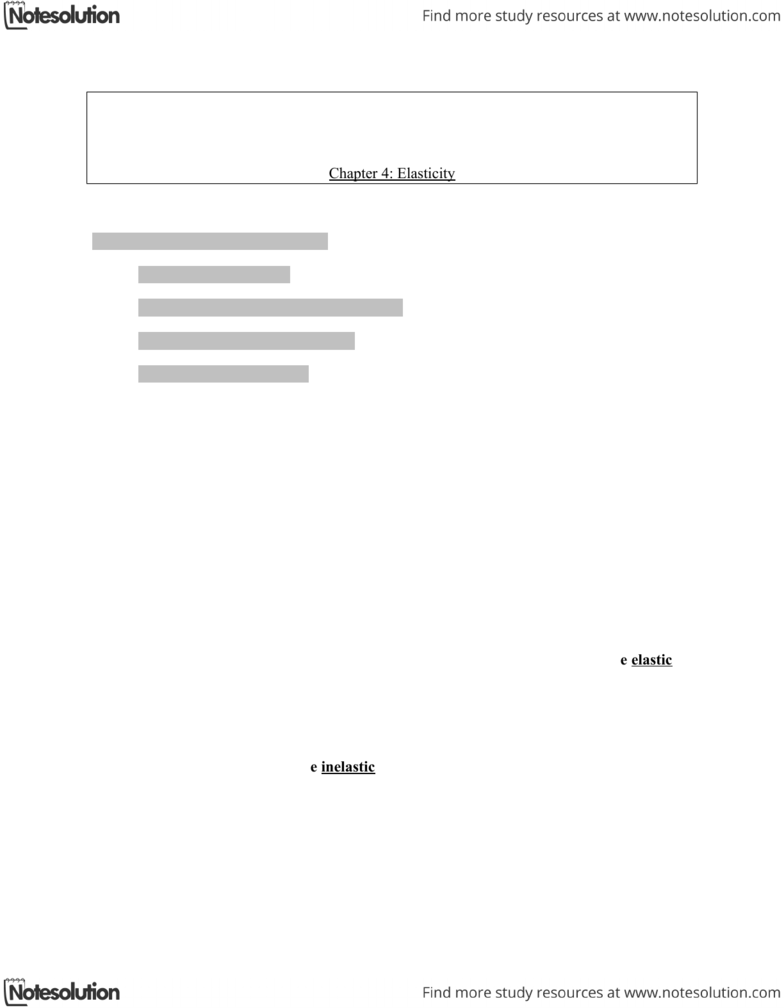# ECON 103 Lecture Notes - Price Elasticity Of Demand, Normal Good

18 views7 pages
School
Simon Fraser University
Department
Economics
Course
ECON 103
Page:
of 7Fraser International College
ECON1034 “Principles of Microeconomics”
Chapter 4: Elasticity
The KEY concepts of this chapter are:
- A slope of a straight line
- Elasticity of demand (interpretation, types)
- Elasticity of supply (interpretation)
- Factors that affect elasticity
Before studying elasticity, it is useful to recall what the slope of a straight line is and how to find
it:
Elasticity is a property of demand and supply. If demand or supply are said to be elastic, it
means That percentage change in Qd or Qs is greater than percentage change in price.
Based on Luxuries commodities.
If demand or supply are said to be inelastic, it means that percentage change in Qs or Qd is
less than percentage change in price. Based on Necessities commodities.
Elasticity is independent of the units of measurement. This is useful because
1
Let’s illustrate elastic and inelastic demand and supply with diagrams:
Elasticity of demand
Price elasticity of demand
Definition: It is a unites-free measurement of the responsiveness of percentage change in Qd /
percentage change in price.
PED refers to responsiveness of change in Qd of a good due to change in its own price.
Inelastic means:Percenatge chage in Qd is less than price.
Elastic means:Percenatge change in Qd is greater than price.
The formula:
% change in Qd (Divided by) % change in P
Example:
2
The range of demand elasticity:
Elasicty Range
Elastic Greater than 1
Inealsric Less than1
Unitary Elastic Equal to 1
Perfectly Inelatic Equal to 0
Perfectly Elastic Equal to Infinity.
Elasticity and slope:
Firm’s revenue and elasticity
Elastic
Price Effect on Revenue
Increase Decrease
Decrese Increase
Inelastic
3

## Document Summary

Before studying elasticity, it is useful to recall what the slope of a straight line is and how to find it: Elasticity is a property of demand and supply. If demand or supply are said to be elastic, it means that percentage change in qd or qs is greater than percentage change in price. If demand or supply are said to be inelastic, it means that percentage change in qs or qd is less than percentage change in price. Based on necessities commodities: elasticity is independent of the units of measurement. Let"s illustrate elastic and inelastic demand and supply with diagrams: Definition: it is a unites-free measurement of the responsiveness of percentage change in qd / percentage change in price. Ped refers to responsiveness of change in qd of a good due to change in its own price. Inelastic means:percenatge chage in qd is less than price. Elastic means:percenatge change in qd is greater than price.